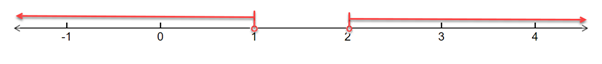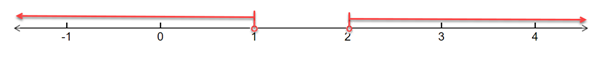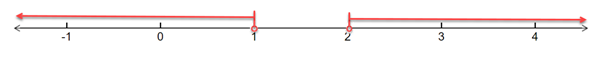# To solve the below inequality in terms of intervals and illustrate the solution set on the real number line - ( x − 1 ) ( x − 2 ) &gt; 0### Single Variable Calculus: Concepts...

4th Edition
James Stewart
Publisher: Cengage Learning
ISBN: 9781337687805### Single Variable Calculus: Concepts...

4th Edition
James Stewart
Publisher: Cengage Learning
ISBN: 9781337687805

#### Solutions

Chapter A, Problem 17E
To determine

## To solve the below inequality in terms of intervals and illustrate the solution set on the real number line -   (x−1)(x−2)>0

Expert Solution

The solution of the inequality is x<1 and x>2 and the solution set on a real number line -### Explanation of Solution

Given: Inequality: (x1)(x2)>0

Formula Used:

An inequality compares two values, showing if one is less than, greater than, or simply not equal to another value.

Real number line is the line whose points are the real numbers.

Calculation:

Given: Inequality equation is (x1)(x2)>0

To solve the above inequalities, we need to find the different intervals for which the inequality gives a value greater than 0 .

When x<1 :

(x1) is negative and (x2) is negative.

Thus, (x1)(x2)>0

So, one of the solutions is x<1

When 1<x<2 :

(x1) is positive and (x2) is negative.

Thus, (x1)(x2)<0

So, 1<x<2 cannot be one of the solutions.

When x>2 :

(x1) is positive and (x2) is positive.

Thus, (x1)(x2)>0

So, one of the solutions is x>2 ;

Thus, combining both the solutions, we have:

x<1 and x>2

Drawing the above inequality on a real number line, we have:Conclusion:

Hence, the solution of the inequality is x<1 and x>2 and the solution set on a real number line -### Have a homework question?

Subscribe to bartleby learn! Ask subject matter experts 30 homework questions each month. Plus, you’ll have access to millions of step-by-step textbook answers!